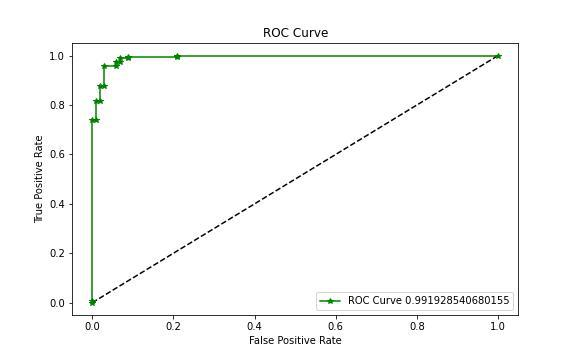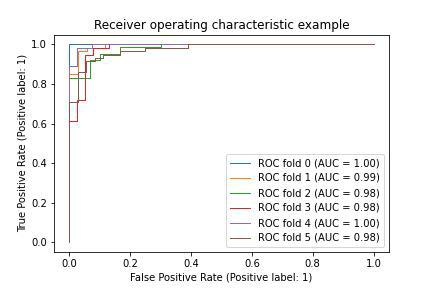Open in App
Not now

# Receiver Operating Characteristic (ROC) with Cross Validation in Scikit Learn

• Difficulty Level : Hard
• Last Updated : 03 Mar, 2023

In this article, we will implement ROC with Cross-Validation in Scikit Learn. Before we jump into the code, let’s first understand why we need ROC curve and Cross-Validation in Machine Learning model predictions.

## Receiver Operating Characteristic Curve (ROC Curve)

To understand the ROC curve one must be familiar with terminologies such as True Positive, False Positive, True Negative, and False Negative. ROC curve is a pictorial or graphical plot that indicates a False Positive vs True Positive relation, where False Positive is on the X axis and True Positive is on the Y axis. In this context, the False Positive rate is denoted as Specificity and the True Positive rate is denoted as Sensitivity.

```Sensitivity = TP/(TP+FN)

Specificity = TN/(TN+FP)```

The top left corner of the ROC curve denotes the ideal point, where the False Positive Rate is 0 and the True Positive Rate is 1. You don’t usually get 1, but a score close to 1 is considered to be a good score.ROC curve can be used as evaluation metrics for the Classification based model. It works well when the target classification is Binary.

## Cross Validation

In Machine Learning splitting the dataset into training and testing might be troublesome sometimes. Cross Validation is a technique using which we select the batches of the different training sets and fit them into the model. This in return helps in generalizing the model and is less prone to overfitting. The commonly used Cross Validation methods are KFold, StratifiedKFold, RepeatedKFold, LeaveOneGroupOut, and GroupKFold.

We shall now implement the cross-validation technique to understand the ROC curve on different samples of the dataset.

## Receiver Operating Characteristic (ROC) with Cross-Validation in Scikit Learn

Before we proceed to implement the code, make sure you have downloaded the sklearn Python module.

`pip install -U scikit-learn`

### Import the required libraries

Here we will import some useful Python libraries like NumPy, Matplotlib, SKlearn for performing complex computational tasks in a few lines of code.

## Python3

 `import` `numpy as np``import` `matplotlib.pyplot as plt` `from` `sklearn ``import` `datasets``from` `sklearn.metrics ``import` `roc_curve, auc,roc_auc_score``from` `sklearn.metrics ``import` `RocCurveDisplay``from` `sklearn.model_selection ``import` `train_test_split``from` `sklearn.linear_model ``import` `LogisticRegression``from` `sklearn.model_selection ``import` `KFold`

SKlearn provides various toy datasets from which we are loading breast_cancer dataset for our article.

## Python3

 `data ``=` `datasets.load_breast_cancer()``X ``=` `data.data``y ``=` `data.target` `print``(X.shape)``print``(y.shape)`

Output:

```(569, 30)
(569,)```

### Define The Cross Validation and Model

In our case, we shall use KFold cross-validation and Logistic Regression since the data end target is Binary Classification.

## Python3

 `cross_val ``=` `KFold(n_splits``=``6``, random_state``=``42``, shuffle``=``True``)``model ``=` `LogisticRegression()`

### Initialize True Positive Rate and Area Under Curve

Since we are using Cross Validation, we will have different samples of training sets. So we will define the mean False Positive rate, True Positive Rate, and Area under Curve as a list or array.

## Python3

 `tprs, aucs ``=` `[], []``mean_fpr ``=` `np.linspace(``0``, ``1``, ``100``)`

### Plot ROC Curve for every Cross Validation Split

Sklearn provides ROC Curve display metrics that take in the model and testing data as the argument to calculate the ROC curve on the given dataset. True positive and Area Under curve is updated on each split.

## Python3

 `fig, ax ``=` `plt.subplots()``for` `index, (train, test) ``in` `enumerate``(cross_val.split(X, y)):``    ``model.fit(X[train], y[train])``    ``plot ``=` `RocCurveDisplay.from_estimator(``        ``model, X[test], y[test],``        ``name``=``"ROC fold {}"``.``format``(index),``        ``ax``=``ax,``    ``)``    ``interp_tpr ``=` `np.interp(mean_fpr, plot.fpr, plot.tpr)``    ``interp_tpr[``0``] ``=` `0.0``    ``tprs.append(interp_tpr)``    ``aucs.append(plot.roc_auc)` `ax.``set``(``    ``xlim``=``[``-``0.05``, ``1.05``],``    ``ylim``=``[``-``0.05``, ``1.05``],``    ``title``=``"Receiver operating characteristic with CV"``,``)``plt.savefig(``"roc_cv.jpeg"``)`

Output:My Personal Notes arrow_drop_up